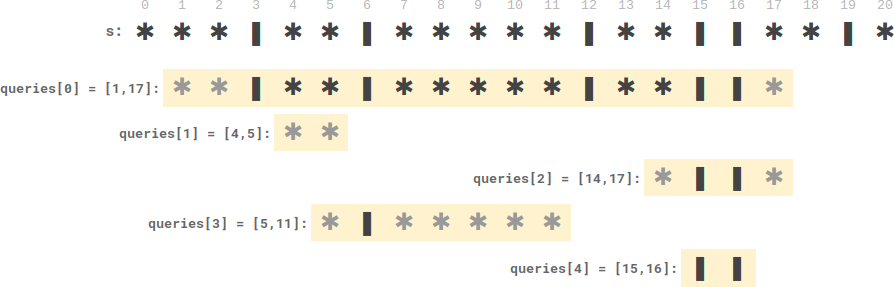# LeetCode Plates Between Candles Solution

There is a long table with a line of plates and candles arranged on top of it. You are given a 0-indexed string `s` consisting of characters `'*'` and `'|'` only, where a `'*'` represents a plate and a `'|'` represents a candle.

You are also given a 0-indexed 2D integer array `queries` where `queries[i] = [lefti, righti]` denotes the substring `s[lefti...righti]` (inclusive). For each query, you need to find the number of plates between candles that are in the substring. A plate is considered between candles if there is at least one candle to its left and at least one candle to its right in the substring.

• For example, `s = "||**||**|*"`, and a query `[3, 8]` denotes the substring `"*||**|"`. The number of plates between candles in this substring is `2` (at indices `6` and `7`), as each of the two plates has at least one candle in the substring to its left and right.

Return an integer array `answer` where `answer[i]` is the answer to the `ith` query.

Example 1:Input: s = "**|**|***|", queries = [[2,5],[5,9]]

Output: [2,3]

Explanation:

• queries has two plates between candles.
• queries has three plates between candles.

Example 2:Input: s = "***|**|*****|**||**|*", queries = [[1,17],[4,5],[14,17],[5,11],[15,16]]

Output: [9,0,0,0,0]

Explanation:

• queries has nine plates between candles.
• The other queries have zero plates between candles.

Constraints:

• `3 <= s.length <= 105`
• `s` consists of `'*'` and `'|'` characters.
• `1 <= queries.length <= 105`
• `queries[i].length == 2`
• `0 <= lefti <= righti < s.length`

## Solution

To find the number of plates for each query `(qleft, qright)`, we set up an array `candles` to store the candles' indices, so that we could later do basic arithmetic on the indices to find the number of plates. First, we need to find the outside candles' indices in the input `s`, this can be done via binary search in `candles`. We will find `left_pos` and `right_pos` indicating the outside candle's position in `s`. Then, We know that the number of plates is given by the interval between the two bounding candles subtracted by the number of candles in between. With the indices `left_pos` and `right_pos`, we can derive the number of plates to be `(candles[right_pos] - candles[left_pos]) - (right_pos - left_pos)`.

#### Implementation

``````1def platesBetweenCandles(s, queries):
2  candles = []
3  for i in range(len(s)):
4      if s[i] == '|': candles.append(i)
5
6  res = []
7  for qleft, qright in queries:
8      left_pos, right_pos = -1, -1
9
10      # 1. find index of first candle that comes after qleft
11      left, right = 0, len(candles)-1
12      while left <= right:
13          mid = (left+right) // 2
14          if candles[mid] >= qleft:
15              right = mid - 1
16              left_pos = mid
17          else:
18              left = mid + 1
19
20      # 2. find index of last candle that comes before qright
21      left, right = 0, len(candles)-1
22      while left <= right:
23          mid = (left+right) // 2
24          if candles[mid] <= qright:
25              left = mid + 1
26              right_pos = mid
27          else:
28              right = mid - 1
29
30      # result = range between two outermost candles - candle count in between
31      if (left_pos != -1) & (right_pos!= -1) & (right_pos > left_pos):
32          res.append((candles[right_pos] - candles[left_pos]) - (right_pos - left_pos))
33      else:
34          res.append(0)
35  return res``````